# AP Calculus BC : Substitution of Variables (by parts and simple partial fractions)

## Example Questions

### Example Question #11 : Fundamental Theorem Of Calculus And Techniques Of Antidifferentiation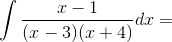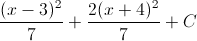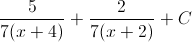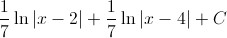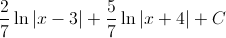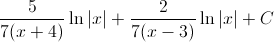Explanation:

In order to evaluate this integral, we will need to use partial fraction decomposition.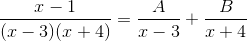Multiply both sides of the equation by the common denominator, which is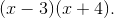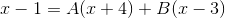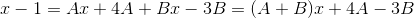This means that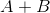must equal 1, and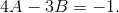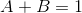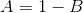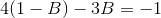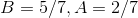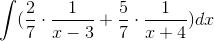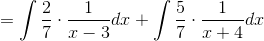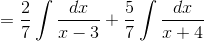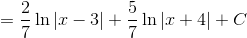The answer is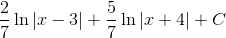.

### Example Question #1 : Substitution Of Variables (By Parts And Simple Partial Fractions)

Integrate: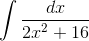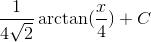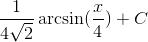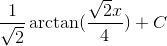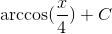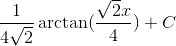Explanation:

To integrate, we must first make the following substitution: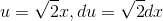Rewriting the integral in terms of u and integrating, we get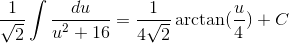The following rule was used to integrate: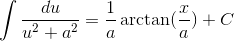Finally, we replace u with our original x term: